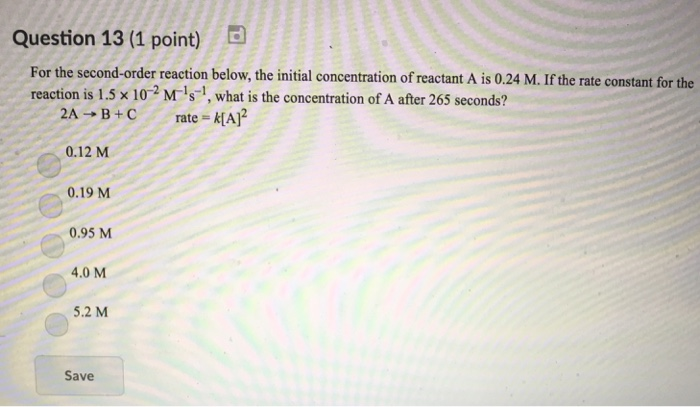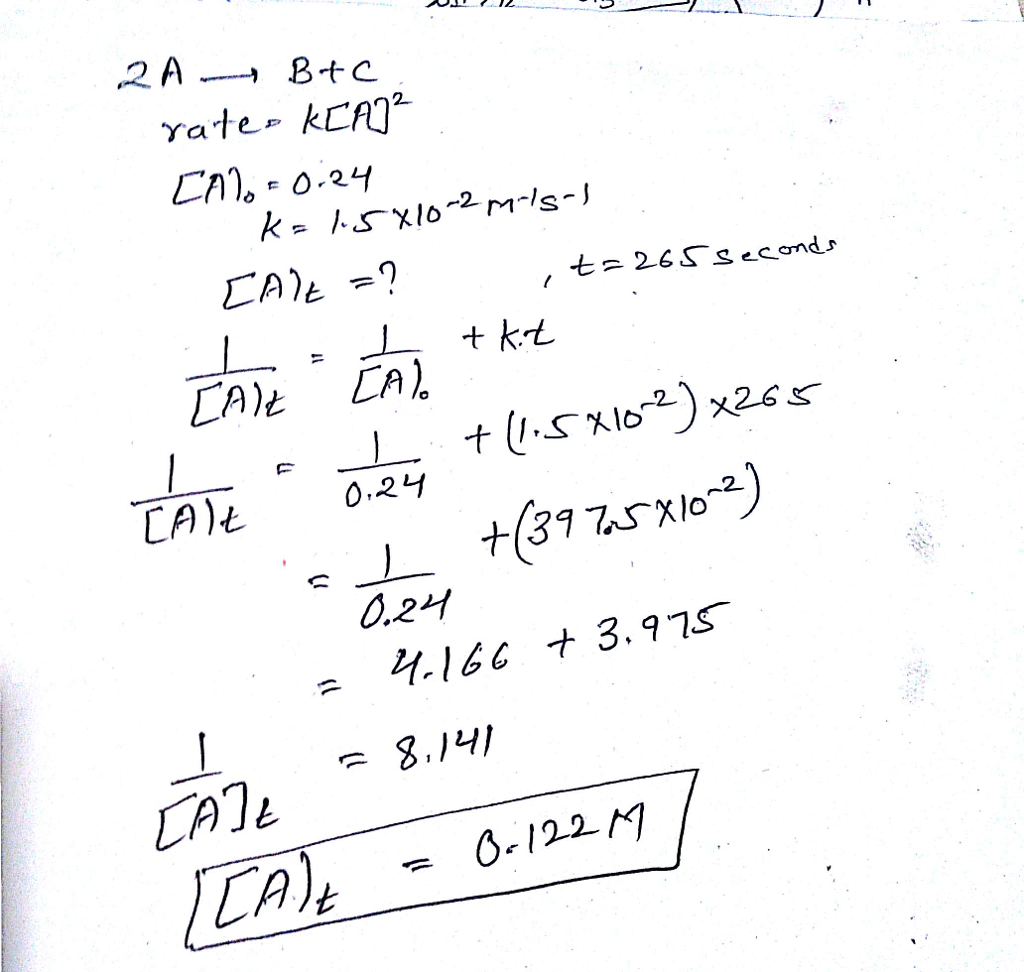# Question & Answer: For the second-order reaction below, the initial concentration of reactant A is 0.24 M. If the…..For the second-order reaction below, the initial concentration of reactant A is 0.24 M. If the rate constant for the reaction is 1.5 times 10^-2 M^-1 s^-1, what is the concentration of A after 265 seconds? 2A rightarrow B + C rate = k[A]^2 0.12 M 0.19 M 0.95 M 4.0 M 5.2 M Save